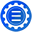If

# If

Description

Conditionally evaluates multiple branches of an expression in which the first branch to evaluate to a true condition returns a string.  There are two completely different syntactical patterns that can be used in this macro.

# Arguments for Syntax Pattern #1

1.IF - Name of the Macro.

2.string-expression-one - The first string to compare

3.Operator - The operator keyword that determines the type of comparison used.

4.string-expression-two - The second string to compare

5.THEN - The constant that indicates that the next value will be returned if the comparison is true

6.result-expression - The value to return if the operator evaluates to true

7.ELSEIF - (Optional) The constant that indicates that the another comparison is beginning.  When used items 7-12 must be provided together

8.string-expression-one - The first string to compare

9.Operator - The operator keyword that determines the type of comparison used

10.string-expression-two - The second string to compare

11.THEN - The constant that indicates that the next value will be returned if the comparison is true

12.result-expression - The value to return if the operator evaluates to true

13.ELSE - (Optional) The constant that indicates that the next value will be returned if no matches have previously been made.  When used items 13-14 must be provided together.

14.default-expression - The value that is returned when no comparison resulted in true.

# Operators

1.EQ or = or == - Indicate string-expression-one must exactly match string-expression-two using string equality tests.

2.NE or != or <> - Indicate string-expression-one must NOT match string-expression-two using string equality tests.

3.GE or GTE or >=- Indicate string-expression-one must sort greater than or equal to string-expression-two using string equality tests.

4.GT or > - Indicate string-expression-one must sort greater than string-expression-two using string equality tests.

5.LE or LTE or <= - Indicate string-expression-one must sort less than or equal to string-expression-two using string equality tests.

6.LT or < - Indicate string-expression-one must sort less than string-expression-two using string equality tests.

7.#EQ or #= or #== - Indicate string-expression-one must have the same numeric value as string-expression-two using numeric equality tests.

8.#NE or #!= or #<> - Indicate string-expression-one must NOT have the same numeric value as string-expression-two using numeric equality tests.

9.#GE or #GTE or #>= - Indicate string-expression-one must be greater than or equal to string-expression-two using numeric equality tests.

10.#GT or #> - Indicate string-expression-one must be greater than string-expression-two using numeric equality tests.

11.#LE or #LTE or #<= - Indicate string-expression-one must be less than or equal to string-expression-two using numeric equality tests.

12.#LT or #< - Indicate string-expression-one must be less than string-expression-two using numeric equality tests.

# Examples

[If|[Attribute|Superhero]|=|Batman|THEN|Gotham]

If the value of [Attribute|Superhero] matches Batman then Gotham is returned otherwise a blank value.

[If|[Attribute|Superhero]|=|Batman|THEN|Gotham|ELSE|Unknown]

If the value of [Attribute|Superhero] matches Batman then Gotham is returned otherwise the word Unknown is returned.

If the value of [Attribute|Superhero] matches Batman then Gotham is returned but if the value of [Attribute|Supervillain] is Joker then Bad is returned otherwise the word Unknown is returned.

[IF|-1|GTE|1|THEN|Y|ELSE|N]

In this case the value -1 sorts higher than 1 when doing a string comparison because of the - character so the result is Y.

[IF|-1|#GTE|1|THEN|Y|ELSE|N]

In this case the value -1 sorts higher than 1 when doing a numeric comparison as one would expect so the result is N.

# Arguments for Syntax Pattern #2

1.If - Name of the Macro.

2.String A - The first string to compare.

3.String B - The second string to compare.

4.Result if True - String to return if the two strings match.

5.Result if False - String to return if the two strings do not match.  If not supplied, string A will be returned.

Examples

[If|[Attribute|Superhero]|Batman|The superhero is Batman.]

If the value of [Attribute|Superhero] matches Batman then "The superhero is Batman." is returned.

If the value of [Attribute|Superhero] does not match Batman then the value of [Attribute|Superhero] is returned.

[If|[Attribute|Superhero]|Batman|The superhero is Batman.|The superhero is not Batman.]

If the value of [Attribute|Superhero] matches Batman then "The superhero is Batman." is returned.

If the value of [Attribute|Superhero] does not match Batman then "The superhero is not Batman." is returned.